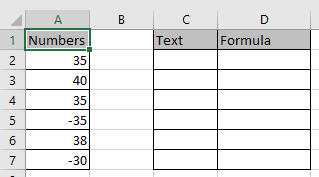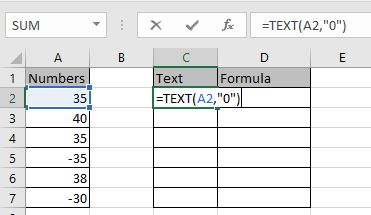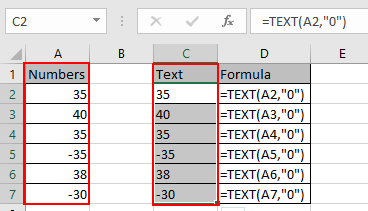# How to Convert Numbers To Text in Excel

In this article, we will learn about how to convert numbers to text using the TEXT function in Excel.

In simple words, the Excel TEXT function lets you convert the number to text and we will do the same in this article.
Syntax

=TEXT (value, format_text)

Let’s use this function on the numbers shown below.Convert the value in the A2 cell.
Use the formula in C2 cell

=TEXT (A2, “0”)

“0”: returns the general text format.Press Enter and copy the formula in remaining cells using Ctrl + DAs you can see we got numbers as text output because the significance number always varied.

I hope you understood how to Convert Numbers To Text in Excel. Explore more articles on Excel functions here. Please feel free to state your query or feedback for the above article.

Related Articles:

How to Use TEXT Function in Excel

How to Convert date to text in Excel

How to Convert Text To Number in Excel

How to Convert Date and Time from GMT to CST in Excel

How to Convert month name to serial number in Excel

How to Convert Excel Column Number To Letter in Excel

How To Convert Column Letter to Number in Excel

Popular Articles:

50 Excel Shortcuts to Increase Your Productivity

How to use the VLOOKUP Function in Excel

How to use the COUNTIF function in Excel

How to use the SUMIF Function in Excel

Terms and Conditions of use

The applications/code on this site are distributed as is and without warranties or liability. In no event shall the owner of the copyrights, or the authors of the applications/code be liable for any loss of profit, any problems or any damage resulting from the use or evaluation of the applications/code.